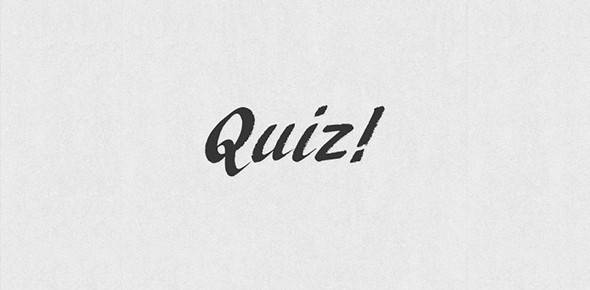# Chapter 7 Practice Quiz Hl - Period 6 - Friday, 4/17/20

5 Questions | Total Attempts: 18SettingsArchbishop Wood High School Physics 9 HL – Period 6 Date: 4/17/20 Chapter 7 Practice Quiz – Using Vectors: Motion and Force Ms. Antes DIRECTIONS: &nb

• 1.
Which force always opposes the motion of an object?
• A.

Gravity

• B.

Normal

• C.

Parallel

• D.

Friction

• 2.
An example of a scalar quantity is:
• A.

Velocity.

• B.

Force.

• C.

Speed.

• D.

Acceleration.

• 3.
Projectiles travel the greatest distance horizontally when the launch angle is:
• A.

10°

• B.

25°

• C.

45°

• D.

60°

• 4.
The normal force is:
• A.

The force acting to always oppose motion.

• B.

The force acting perpendicular to the surface.

• C.

The force from gravity.

• D.

The force acting parallel to the surface.

• 5.
An example of a vector quantity is:
• A.

Temperature.

• B.

Length.

• C.

Velocity.

• D.

Mass.Back to top